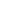# Free CBSE Class 3 Maths Addition Worksheets

## Sample CBSE Class 3 Maths Addition Worksheet Questions

1.

2.

3.

4.

Fill in the missing number:

 TH H T O 6 9 ____ 3 + 3 2 1 ____ _____ ____ 6 7
5.

Match the following:

 a).  4660 + 1 1).  450 b).  630 + 0 2).  Additive property of zero c).  5000 + 400 + 200 + 10 3).  3007 d).  8 Thousands + 4 HUndreds + 2 Tens + 1 Ones 4).  2360 e).  300 5).  4661 f).  100 more than 999 6).  8421 g).  2350 + 10 7).  1099 h).  450 + 00 8).  630 i).  3000 + 7 9).  200 + 100 j).  1234 + 0 = 0 + 1234 10). 5421
6.
Add the following: Smallest 3 digit numbers and 1000
7.
Add the following: Largest 3 digit number and 1
8.
Add the following: Smallest four digit number and 0
9.
State True or False: 134 + 300 = 200 + 134
1. true 2. false
10.
State True or False: 25 more than 75 is 750
1. true 2. false

Worksheets by UrbanPro

Our worksheets are designed to help students explore various topics, practice skills and enrich their subject knowledge, to improve their academic performance. Designed by Experts who have extensive experience and expertise in teaching a subject, these worksheets will improve your child's problem-solving skills and subject knowledge in a fun and interactive manner.
Check out our free customized worksheets across school boards, grades, subjects and levels of subject knowledge. You can download, print and share these worksheets with anyone, anywhere, anytime!

Get a custom worksheet to practice!

Select your topic & see the magic.

subjectSelect Chapter(s)

Chapters & Subtopics